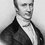# Proving the impossibilityuhh

Yesterday i was puzzling with some problems related to fractions ,for example:- Find out the coprime possitive integers (a,b) such that when a is divided by b yields the fraction 0..688888888....... Problem can be easily solved as follows:- $let x=0.68888888888....-(1)$ Multiplying both sides by 100:- $100x=68.88888888.....-(2)$ Subtracting equation one from equation two:- $99x=68.2$ On canceling comen divisers:- $x=\frac{31}{45}$ Since 31 and 45 are coprime so answer is (31,45) Mathod can be used to convert all rational fractions into the form $\frac{a}{b}$ After solving five or six such problems by using this mathod i tried to convert 0.99999999.... into the form a÷b but got a contradiction as follows:- $let x=0.999999...........-(1)$ Multiplying both sides by 10:- $10x=9.99999999.......-(2)$ Subtracting (1) from (2):- $9x=9 ==> x=1$ But from equation (1) x=0.99999..... So does this means that 0.99999....=1 . . Well i think this could be probebly because 0.99999.... is an irrational number (i think so),but problem is that i am not much fimiler with mumber theory so i don't know any mathod to prove that this number is irrational...what do you think about it..

Sorry for all grammer and spelling mistakes i am not good in english :pNote by Aman Sharma
6 years, 10 months ago

This discussion board is a place to discuss our Daily Challenges and the math and science related to those challenges. Explanations are more than just a solution — they should explain the steps and thinking strategies that you used to obtain the solution. Comments should further the discussion of math and science.

When posting on Brilliant:

• Use the emojis to react to an explanation, whether you're congratulating a job well done , or just really confused .
• Ask specific questions about the challenge or the steps in somebody's explanation. Well-posed questions can add a lot to the discussion, but posting "I don't understand!" doesn't help anyone.
• Try to contribute something new to the discussion, whether it is an extension, generalization or other idea related to the challenge.

MarkdownAppears as
*italics* or _italics_ italics
**bold** or __bold__ bold
- bulleted- list
• bulleted
• list
1. numbered2. list
1. numbered
2. list
Note: you must add a full line of space before and after lists for them to show up correctly
paragraph 1paragraph 2

paragraph 1

paragraph 2

[example link](https://brilliant.org)example link
> This is a quote
This is a quote
    # I indented these lines
# 4 spaces, and now they show
# up as a code block.

print "hello world"
# I indented these lines
# 4 spaces, and now they show
# up as a code block.

print "hello world"
MathAppears as
Remember to wrap math in $$ ... $$ or $ ... $ to ensure proper formatting.
2 \times 3 $2 \times 3$
2^{34} $2^{34}$
a_{i-1} $a_{i-1}$
\frac{2}{3} $\frac{2}{3}$
\sqrt{2} $\sqrt{2}$
\sum_{i=1}^3 $\sum_{i=1}^3$
\sin \theta $\sin \theta$
\boxed{123} $\boxed{123}$

Sort by:

Anytime you have a repeating decimal, it's a rational number. So, 0.9999999.... etc is pretty rational to me.

Another example:

$0.05882352941176470588235294117647058823529....$

looks irrational, but it actually repeats (look CAREFULLY). This one is $1/17$.

- 6 years, 10 months ago

Well reccuring decimal numbers are always rational i read it somewhere..........but sir i am realy confused with 0.9999999....'s case, i am realy intrested in its solutions..i am trying to find numbers a and b such that a/b=0.99999........ I also tried to solve it by converting number into infinite series but in vain

Also number given by you is aldo rational i solved it by mathod of differences

- 6 years, 10 months ago

hard works makes man perfect

- 6 years, 3 months ago

See.. no. Is irrational only when it is neither recurring nor repeating....like

.99999999 or .12367456456456

so both these no. Are rational and can be convert into a:b...

- 6 years, 10 months ago

Can you convert 0.99999......... into a:b

- 6 years, 10 months ago